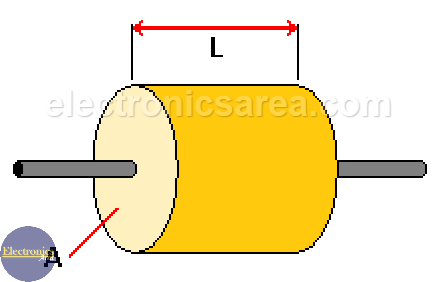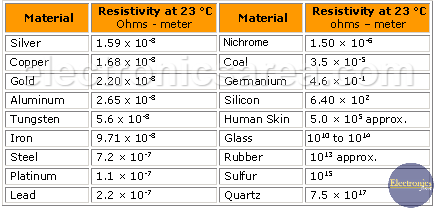# What is Electrical Resistivity?

Electrical resistivity [ρ] (rho) is a characteristic of materials and has ohm-meter unit. Resistivity indicates how much the material opposes the flow of electric current.

The resistivity [ρ] (rho) has the formula: ρ = R*A/L. where:

• A is the cross sectional area (m2).
• ρ is the resistivity, (ohms-meter).
• L is the length of the material (meters).
• R is the value of the electrical resistance (ohms).From the previous formula it is deduced that the resistor value depends on its construction, the resistivity (material with which it was manufactured), its length and its cross sectional area.

Clearing “R” (resistor value) from the previous formula we obtain: R = ρ*L/A.

If we analyze the formula, it follows that:

• The greater the length and the smaller the cross-sectional area of ​​the element, the greater the resistance.
• The smaller the length and the greater the cross-sectional area of ​​the element, the lower the resistance.

The following table shows typical values ​​of resistivity of various materials at 23° C:## Resistivity depends on temperature

Resistivity does not remain constant with the variation of the temperature. Resistivity of metals increases if the temperature increases, unlike semiconductors, where this value decreases.## What is conductivity?

Conductivity or Electrical Conductivity is a measure of a material’s ability to conduct an electric current. Conductivity (σ) [sigma] is the inverse of resistivity and its formula is: σ = 1/ρ = 1/(R*A/L) = L/R*A.

Example 1

The resistance of 25 meter copper wire with 3 mm2 of cross sectional area can be calculated as:

(Copper wire has a resistivity of 1.7 x 10-8 Ω m).

R = ρ * L / A = (1.7 x 10-8 Ω m) (25 m) / ((3.00 mm2)(10-6 m2/mm2)) = = 0.1417 Ω.

note: 1 mm2 = 1 x 10-6 m2.

Example 2

The copper wire above is reduced to a 0.50 mm2 cross-sectional area. The increase in resistance can be calculated to:

R = ρ * L / A. = (1.7 x 10-8 Ω m) (25m) / ((0.50 mm2)(10-6 m2/mm2)) = 0.85 Ω.

note: 1 mm2 = 1 x 10-6 m2.

• 22
•
•
•
•
•
•
•
•
22
Shares
•
22
Shares
• 22
•
•
•
•
•
•
•